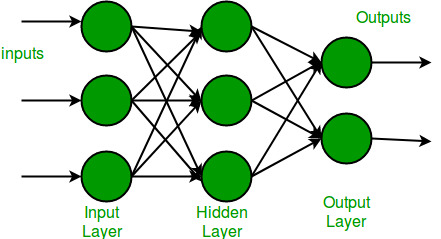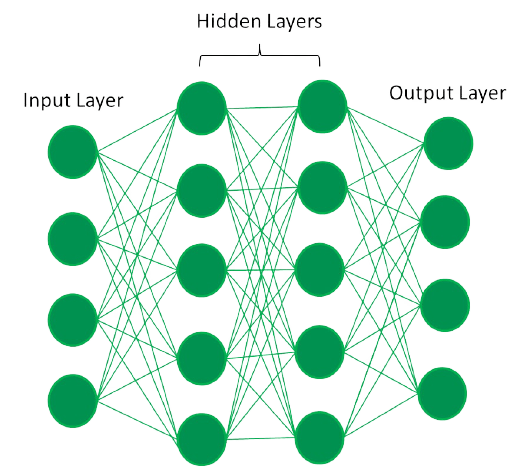Open In App

# Difference between a Neural Network and a Deep Learning System

Since their inception in the late 1950s, Artificial Intelligence and Machine Learning have come a long way. These technologies have gotten quite complex and advanced in recent years. While technological advancements in the Data Science domain are commendable, they have resulted in a flood of terminologies that are beyond the understanding of the average person.

There are so many companies of all sizes out there that use these technologies viz. AI and ML in their day-to-day applications. Yet many have trouble distinguishing between their vast terminologies. Most people even use the terms “Machine Learning”, “Deep Learning” and “Artificial Intelligence” interchangeably.

The reason behind this confusion is that although they have so many different names for different concepts – most of them are deeply entwined with one another and share similarities. Even so, each of these terminologies in itself is unique and useful in its way.

Now, let’s talk about Neural Networks and Deep Learning systems individually before we can see their differences!

## What is a Neural Network?• Neural Networks are inspired by the most complex object in the universe – the human brain. Let us understand how the brain works first. The human brain is made up of something called Neurons. A neuron is the most basic computational unit of any neural network, including the brain.
• Neurons take input, process it, and pass it on to other neurons present in the multiple hidden layers of the network, till the processed output reaches the Output Layer.
• Neural networks are algorithms that can interpret sensory data via machine perception and label or group the raw data. They are designed to recognize numerical patterns contained in vectors that need to transform all real-world data (images, sounds, text, time series, etc.)
• In its simplest form, an Artificial Neural Network (ANN) has only three layers – the input layer, the output layer, and a hidden layer.

## What is Deep Learning?

Now that we have talked about Neural Networks, let’s talk about Deep Learning.

Deep learning, also known as hierarchical learning, is a subset of machine learning in artificial intelligence that can mimic the computing capabilities of the human brain and create patterns similar to those used by the brain for making decisions. In contrast to task-based algorithms, deep learning systems learn from data representations. It can learn from unstructured or unlabeled data.

### What is a Deep Learning System?• A neural network with multiple hidden layers and multiple nodes in each hidden layer is known as a deep learning system or a deep neural network. Deep learning is the development of deep learning algorithms that can be used to train and predict output from complex data.
• The word “deep” in Deep Learning refers to the number of hidden layers i.e. depth of the neural network. Essentially, every neural network with more than three layers, that is, including the Input Layer and Output Layer can be considered a Deep Learning Model.

## Table of Differences between a Neural Network and a Deep Learning System

Now that we have talked about Neural Networks and Deep Learning Systems, we can move forward and see how they differ from each other!

## Architecture

### Neural Network architectures in detail:

• Feedforward Neural Networks – This is the most common type of neural network architecture, with the first layer being the input layer and the last layer being the output layer. All middle layers are hidden layers.
• Recurrent Neural Network – This network architecture is a series of ANNs in which connections between nodes form a directed graph along a temporal sequence. Therefore, this type of network exhibits dynamic behavior over time.
• Symmetrically Connected Neural Networks – These are similar to recurrent neural networks, with the only difference being that the connections between units are symmetric in symmetrically connected neural networks (i.e. same weight n both directions).

### Deep Learning model architectures in detail:

• Unsupervised Pre-Trained Network – As the name implies, this architecture is pre-trained based on past experiences and does not require formal training. These include Autoencoders and Deep Belief networks
• Convolutional Neural Network – This is a deep learning algorithm that can take an input image, assign meaning to various objects in the image (learnable weights and biases), and distinguish between these objects.
• Recursive Neural Network – This is created by recursively applying the same set of weights to a structured input and passing a topological structure to generate a structured prediction over a scalar prediction on a variable-size input structure.

## Structure

### Neural Network structures in detail:

A neural network has the following components

• Neurons – A neuron is a mathematical function that attempts to mimic the behavior of a biological neuron. It calculates the weighted average of the data supplied and then sends the data through a nonlinear function, called the logistic function.
• Connections and weightsConnections link a neuron in one layer to another neuron in the same layer or another layer, as the name implies. A weight value is assigned to each connection. The strength of the relationship between the units is represented by a weight. The goal is to lower the weight number in order to diminish the chances of losing weight (error).
• Propagation – In a Neural Network, there are two propagation functions: forward propagation, which produces the “predicted value,” and backward propagation, which delivers the “error value.”
• Learning Rate – Gradient Descent is used to train neural networks. At each iteration, the derivative of the loss function is calculated in reference to each weight value using back-propagation and then subtracted from that weight. The learning rate determines how quickly or slowly the weight values of the model are updated.

### Deep Learning model structures in detail:

A deep learning model has the following components

• Motherboard – The motherboard chips of the deep learning model are usually based on PCI-e lanes.
• Processors – The GPU requirements for Deep Learning models must be decided based on the processor’s core count and cost.
• Random Access Memory (RAM) – Deep learning models require huge amounts of computational power and storage. For this, they need bigger RAMs.
• Power Supply Unit (PSU) – As memory needs grow, it becomes increasingly important to have a big Power Supply Unit capable of handling enormous and complicated Deep Learning functions.

## Conclusion

Because Deep Learning and Neural Networks are so closely related, it’s difficult to tell them apart on the surface. However, you’ve probably figured out that Deep Learning and Neural Networks are not exactly the same thing.

Deep Learning is associated with the transformation and extraction of features that attempt to establish a relationship between stimuli and associated neural responses present in the brain, whereas Neural Networks use neurons to transmit data in the form of input to get output with the help of the various connections.